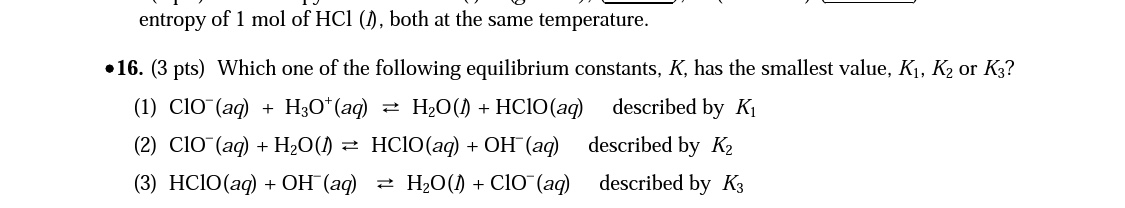# Which one of the following equilibrium constants, K, has the smallest value, K1, K2 or K3 (1) ClO-(aq) + H3O+(aq) ↔ H2O(l) + HClO(aq) described by K1 (2) ClO-(aq) + H2O(l) ↔ HClO(aq) + OH-(aq) described by K2 (3) HClO(aq) + OH-(aq) ↔ H2O(l) + ClO-(aq) described by K1